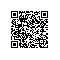# 剑指offer系列之三：在二维数组中查找元素

package com.rhwayfun.offer;

public class MartrixFoundSolution {

public boolean find(int [][] array,int target) {
boolean found = false;
int rows = array.length;
int columns = array.length;
int row = 0;
int column = columns - 1;
while(row < rows && column >=0){
if(array[row][column] == target){
found = true;
break;
}else if(array[row][column] > target){
column--;
}else{
row++;
}
}
return found;
}

public static void main(String[] args) {
int[][] array = new int;
array = new int[]{1,2,8,9};
array = new int[]{2,4,9,12};
array = new int[]{4,7,10,13};
array = new int[]{6,8,11,15};

boolean found = new MartrixFoundSolution().find(array, 7);
System.out.println(found);
}
}使用钉钉扫一扫加入圈子
+ 订阅